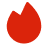# Beaconfire数据科学面试真题

BQ

A/B testing（0）
Statistics（0）
Product Case（0）
Coding（3）
Modeling（0）高频题（0）

A/B testing（0）
Statistics（0）
Product Case（0）
Coding（3）
Modeling（0）高频题（0）
1.LeetCode 1448
2.LeetCode 40
3.LeetCode 394
1. LeetCode 1448
Given a binary tree root, a node X in the tree is named good if in the path from root to X there are no nodes with a value greater than X.

Return the number of good nodes in the binary tree.

Example 1:

```Input: root = [3,1,4,3,null,1,5]
Output: 4
Explanation: Nodes in blue are good.
Root Node (3) is always a good node.
Node 4 -> (3,4) is the maximum value in the path starting from the root.
Node 5 -> (3,4,5) is the maximum value in the path
Node 3 -> (3,1,3) is the maximum value in the path.```
Example 2:

```Input: root = [3,3,null,4,2]
Output: 3
Explanation: Node 2 -> (3, 3, 2) is not good, because "3" is higher than it.```
Example 3:

```Input: root = 
Output: 1
Explanation: Root is considered as good.```

Constraints:

• The number of nodes in the binary tree is in the range [1, 10^5].
• Each node's value is between [-10^4, 10^4].
2. LeetCode 40
Given a collection of candidate numbers (candidates) and a target number (target), find all unique combinations in candidates where the candidate numbers sum to target.

Each number in candidates may only be used once in the combination.

Note: The solution set must not contain duplicate combinations.

Example 1:

```Input: candidates = [10,1,2,7,6,1,5], target = 8
Output:
[
[1,1,6],
[1,2,5],
[1,7],
[2,6]
]
```
Example 2:

```Input: candidates = [2,5,2,1,2], target = 5
Output:
[
[1,2,2],

]
```

Constraints:

• 1 <= candidates.length <= 100
• 1 <= candidates[i] <= 50
• 1 <= target <= 30
3. LeetCode 394
Given an encoded string, return its decoded string.

The encoding rule is: k[encoded_string], where the encoded_string inside the square brackets is being repeated exactly k times. Note that k is guaranteed to be a positive integer.

You may assume that the input string is always valid; there are no extra white spaces, square brackets are well-formed, etc. Furthermore, you may assume that the original data does not contain any digits and that digits are only for those repeat numbers, k. For example, there will not be input like 3a or 2.

The test cases are generated so that the length of the output will never exceed 105.

Example 1:

```Input: s = "3[a]2[bc]"
Output: "aaabcbc"
```
Example 2:

```Input: s = "3[a2[c]]"
Output: "accaccacc"
```
Example 3:

```Input: s = "2[abc]3[cd]ef"
Output: "abcabccdcdcdef"
```

Constraints:

• 1 <= s.length <= 30
• s consists of lowercase English letters, digits, and square brackets '[]'.
• s is guaranteed to be a valid input.
• All the integers in s are in the range [1, 300].